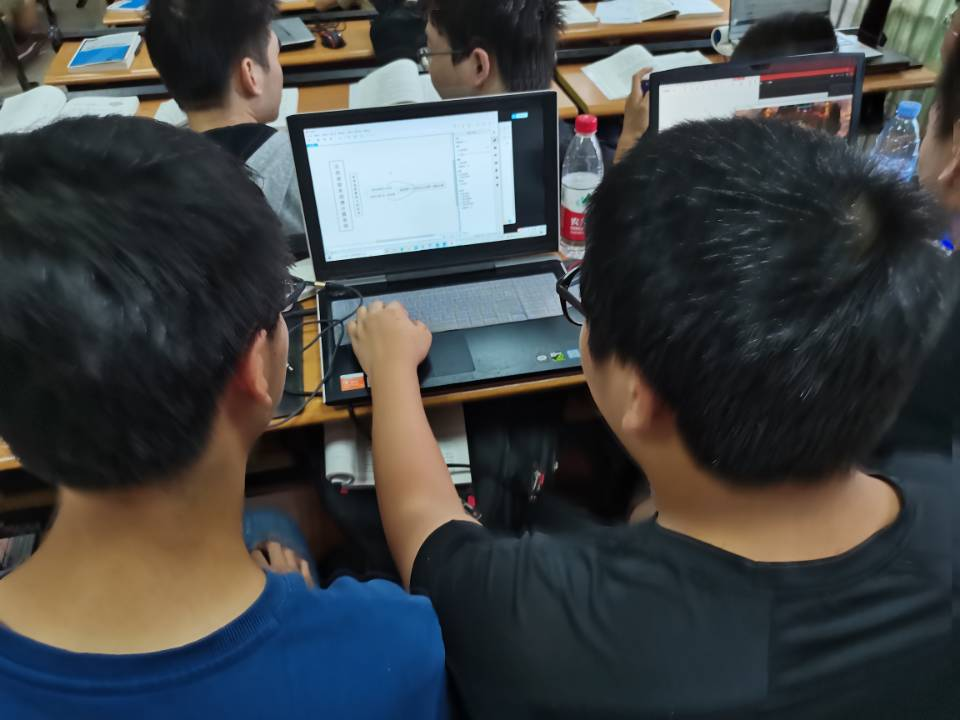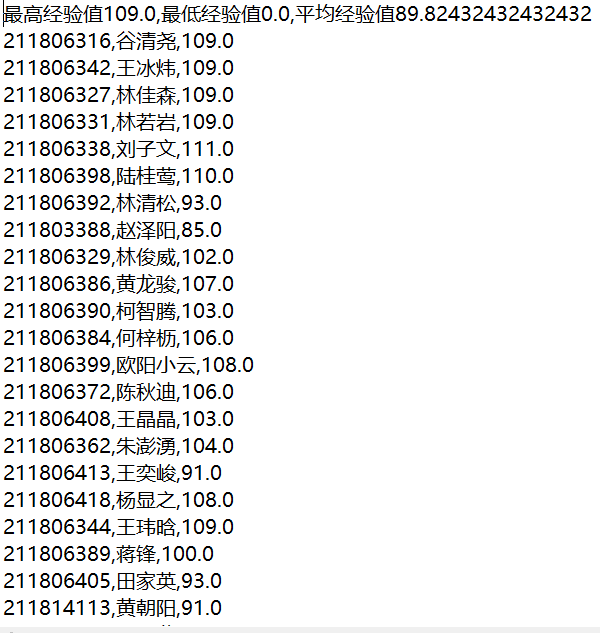# 需求分析&方案设计

## 工作照片## 编码过程

//寻找课堂完成部分题目
for (int i = 0; i < e.size(); i++) {
Element rows_ChildElement = document.select("div[class=interaction-row]").get(i);
if (rows_ChildElement.select("span").get(1).toString().contains("课堂完成")) {
System.out.println(rows_ChildElement.attr("data-url"));
}
}

//计算成绩
public static int getStudentFraction(Elements a,int i) {
int studentfraction;
Element Score = a.get(i).child(3).child(1).child(1);
String Fraction = Score.text();
if (Fraction.contains("尚无评分")||Fraction.contains("批注")) {
studentfraction = 0;
}
else {
Fraction=Fraction.replaceAll("分","").replaceAll(" ","");
studentfraction =  Integer.parseInt(Fraction);
}
return studentfraction;

//写入txt文件
public static void write(ArrayList<Students> StudentList) throws FileNotFoundException {
File file = new File("score.txt");
PrintWriter printWriter = new PrintWriter(new FileOutputStream(file), true);
double sum = 0.0;
double ave = 0.0;
for (int j = 0; j < StudentList.size(); j++) {
sum += StudentList.get(j).getScore();
}
ave = sum / StudentList.size();
Collections.sort(StudentList);
printWriter.println("最高经验值"+StudentList.get(0).getScore()+",最低经验值"+StudentList.get(StudentList.size()-1).getScore()+",平均经验值"+ave);
for (int i = 0; i < StudentList.size(); i++) {
printWriter.println(StudentList.get(i).toString());
}
printWriter.close();
}


## txt文件输出效果